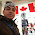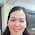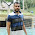## Wednesday, July 20, 2011

### Calculate the CM of a Garments.

Learn Apparel Merchandising, Learn Fashion design.
Dear All,
My this post for these people, who's are still confused regarding the CM (cost of making) of a knit items (garments). To find out the CM of a item you must need the following 06 (six) information at first, as listed below:

i. Monthly total expenditure of your factory with factory rent,commercial cost, electricity bill, water bill, transportation, repairing, worker & stuff wages etc. (8hrs/day) in bangla taka. Suppose - 50,00,000/- tk

ii. Qty of running Machine of your factory of the following month (which total expenditure we have consider here). Suppose - 100 machines

iii. Number of machine to complete the layout for the following Items (which CM we are calculating). Suppose - 25 machines

iv. Production target/capacity of the following items, per hour from the existing layout, excluding alter & reject. Suppose - 200 pcs per hour

v) Total working day of the followings month,(though the house rent,commercial expenses,machine overhauling & some other cost remain same)Suppose- 26 days.

v. If you want to calculate the CM in US\$ (dollar) then pls input present dollar conversation rate BDTk. Suppose - \$1 = 74 taka.

Now you should put the following information in the following form, which you will get here http://cmcostofaknitgarments.blogspot.com/

Otherwise you may follow the below rule:

COST OF MAKING (CM)

= {(Monthly total expenditure of the following factory / 26) / (Qty of running Machine of your factory of the following month) X (Number of machine to complete the layout)} / [{(Production capacity per hr from the existing layout,excluding alter & reject) X 8}] X 12 / (Dollar conversion rate)

= [{(50,00,000 / 26) / ( 100 ) X (25)} / {(200) X 8}] X 12 / 74

= [{192307.7 / (100) X (25) } / 1600] X 12 / 74

= (48,076.9 / 1600) X 12 / 74

= 30.048 X 12 / 74

= 360.58 / 74

= \$4.873 / dozen (this is the making cost (12 pcs) of the following items)

However, normally at present (after starting the new salary scale) in Bangladesh we calculate the CM of any item consider the overhead sewing machine cost 1200 tk to 1400 tk/day that means \$16.216 to \$18.92/per day.

Above is for a non-compliance factory. For the a compliance factory the per day machine cost will be 1800 tk to 2100 tk ( \$24.32 to \$28.37)

SO, if the an items produce 1600 pcs per day using 25 machines then the CM will be

= Overhead machine cost X require machine / produce quantity X 12 / \$74

= 1400 X 25 / 1600 X 12 / 74

= \$3.547/DOZ

Thanks
BIPUL

1.2.thk lot, daniel,0843843.

3.All of ur post really so helpful.
Hope u'll continue it.

Ur regarded,
Md. Matiur Rahman.

1.4.thanks a lot

Ajhar

5.Thanks a lot

Ajhar

6.This comment has been removed by a blog administrator.

7.This comment has been removed by a blog administrator.

8.Bepul bhai thanks for ur great posts

9.Thanks a lot for sharing :)

10.Thanks a lot for sharing this. regards

11.thanks for sharing us such a valuable information

Regards,
Mamun

12.Really helpful & thanks for sharing...

Thank you
Bahalul!

13.Really helpful & thanks for sharing...

Thank you
Bahalul!

14.need sewing machine lay out of a polo shirt

thank you
mamun

15.Its totally outstanding..so helpful...i appreciate you.....

16.am higley appreciate....this is the real merchandising book as you said ...

thanks a lot ...

17.can u send me formula of tee costing

18.Dear Bepul Bhai

i can't understand above mentioned commercial cost please clearly define

Thanks
Regards

19.Great great great

20.Bro pls make the correction

CM = [{(MTE / TWD) / (NRM / NML)} / (PCH x WHD)] x 12 / CR)}

21.(Qty of running Machine of your factory of the following month) X (Number of machine to complete the layout)} it should be
(Qty of running Machine of your factory of the following month) / (Number of machine to complete the layout)}
22.23.